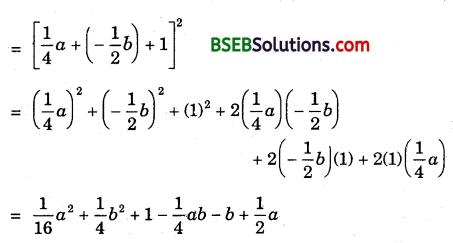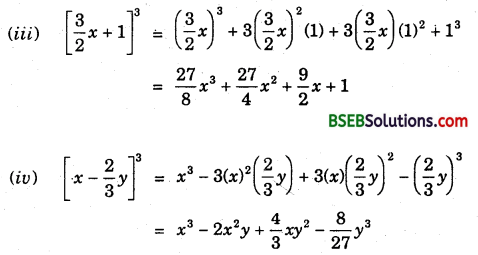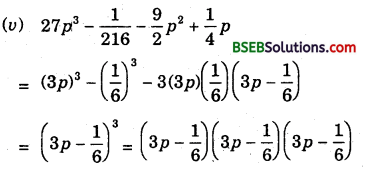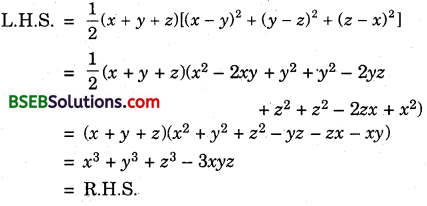# HSSlive: Plus One & Plus Two Notes & Solutions for Kerala State Board

## BSEB Class 9 Maths Chapter 2 Polynomials Ex 2.5 Textbook Solutions PDF: Download Bihar Board STD 9th Maths Chapter 2 Polynomials Ex 2.5 Book AnswersBSEB Class 9 Maths Chapter 2 Polynomials Ex 2.5 Textbook Solutions PDF: Download Bihar Board STD 9th Maths Chapter 2 Polynomials Ex 2.5 Book Answers

BSEB Class 9th Maths Chapter 2 Polynomials Ex 2.5 Textbooks Solutions and answers for students are now available in pdf format. Bihar Board Class 9th Maths Chapter 2 Polynomials Ex 2.5 Book answers and solutions are one of the most important study materials for any student. The Bihar Board Class 9th Maths Chapter 2 Polynomials Ex 2.5 books are published by the Bihar Board Publishers. These Bihar Board Class 9th Maths Chapter 2 Polynomials Ex 2.5 textbooks are prepared by a group of expert faculty members. Students can download these BSEB STD 9th Maths Chapter 2 Polynomials Ex 2.5 book solutions pdf online from this page.

## Bihar Board Class 9th Maths Chapter 2 Polynomials Ex 2.5 Books Solutions

 Board BSEB Materials Textbook Solutions/Guide Format DOC/PDF Class 9th Subject Maths Chapter 2 Polynomials Ex 2.5 Chapters All Provider Hsslive

## How to download Bihar Board Class 9th Maths Chapter 2 Polynomials Ex 2.5 Textbook Solutions Answers PDF Online?

2. Click on the Bihar Board Class 9th Maths Chapter 2 Polynomials Ex 2.5 Answers.
3. Look for your Bihar Board STD 9th Maths Chapter 2 Polynomials Ex 2.5 Textbooks PDF.
4. Now download or read the Bihar Board Class 9th Maths Chapter 2 Polynomials Ex 2.5 Textbook Solutions for PDF Free.

Find below the list of all BSEB Class 9th Maths Chapter 2 Polynomials Ex 2.5 Textbook Solutions for PDF’s for you to download and prepare for the upcoming exams:

## BSEB Bihar Board Class 9th Maths Solutions Chapter 2 Polynomials Ex 2.5

Question 1.
Use suitable identities to find the following products :
(i) (x + 4)(x + 10)
(ii) (x + 8)(x – 10)
(iii) (3x + 4) (3x – 5)
(iv) (y² + 32)(y² – 32)
(v) (3 – 2x)(3 + 2x)
Solution:
(i) (x + 4)(x + 10) = x² + (4 + 10)x + 4 x 10
= x² + 14x + 40

(ii) (x + 8)(x – 10) = x² + (8 – 10)x + 8 x – 10
= x² – 2x – 80

(iii) (3x + 4)(3x – 5) = 3x(3x – 5) + 4(3x – 5)
= 3x × 3x – 3x + 5 + 4 x 3x – 4 x 5
= 9x² – 15x + 12x – 20
= 9x² – 3x – 20

(iv) (y² + 32)(y² – 32)
= (y²)² = (32)² = y4 – 94

(v) (3 – 2x)(3 + 2x) = (3)² – (2x)²
= 9 – 4x²

Question 2.
Evaluate the following products without multiplying directly :
(i) 103 x 107
(ii) 95 x 96
(iii) 104 x 96
Solution:
(i) 103 x 107 = (100 + 3 x 100 + 7)
= (100)² + (3 + 7)(100) + 3 x 7
= 100 x 100 + (10)(100) + 21
= 10000 + 1000 + 21 = 11021

(ii) 95 x 96 = (100 – 5)(100 – 4)
= (100)² + (- 5 – 4 x 100) + (- 5)(- 4)
= 100 x 100 + (- 9)(100) + 20
= 10000-900 + 20 = 9120

(iii) 104 x 96 = (100 + 4)(100 – 4)
= (100)² – (4)²
= 10000 – 16
= 9984

Question 3.
Factorise the following using appropriate identities:
(i) 9x² + 6xy + y²
(ii) 4y² – 4y + 1
(iii) x² – 𝑦2100
Solution:
(a) 9x² + 6xy + y² = (3x)² + 2(3x)(y) + (y)²
= (3x + y)²
= (3x + y)(3x + y)

(b) 4y² – 4y + 1 = (2y)² – 2(2y)(1) + (1)²
= (2y – 1)² = (2y – 1)(2y – 1)

(c) x² – 𝑦2100 = (x)² – (𝑦10)²
= (x – 𝑦10)(x + 𝑦10)

Question 4.
Expand each of the following, using suitable identifies:
(i) (x + 2y + 4z)²
(ii) (2x – y + z)²
(iii) (- 2x + 3y + 2z)²
(iv) (3a – 7b – c)²
(v) (- 2x + 5y – 3z)²
(vi) [14𝑎−12𝑏+1]2
Solution:
(i) (x + 2y + 4z)²
= x² + (2y)² + (4z)² + 2(x)(2y) + 2(2y)(4z) + 2(4z)(x)
= x² + 4y² + 16z² + 4xy + 16yz + 8zx

(ii) (2x – y + z)²
= [2x + (- y) + z]²
= (2x)² + (- y)² + z² + 2(2x)(- y) + 2(-y)(z) + 2(z)(2x)
= 4x² + y² + z² – 4xy – 2yz + 4zx

(iii) (- 2x + 3y + 2z)²
= [(- 2x) + 3y + 2z]²
= (- 2x)² + (3y)² + (2z)² + 2(- 2x)(3y) + 2(3y)(2z) + 2(2z)(- 2x)
= 4x² + 9y² + 4z² – 12xy + 12yz – 8zx

(iv) (3a – 7b – c)²
= [3a + (- 7b) + (- c)]²
= (3a)² + (- 7b)² + (- c)² + 2(3a)(- 7b) + 2(- 7b)(- c) + 2(- c)(3a)
= 9a² + 49b² + c² – 42ab + 146c – 6ca

(v) (- 2x + 5y – 3z)²
= [(- 2x)² + 5y + (- 3z)]²
= (- 2x)² + (5y)² + (- 3z)² + 2(- 2x)(5y) + 2(5y)(- 3z) + 2(- 3z)(- 2x)
= 4x² + 25y² + 9z² – 20xy – 30yz + 12zx

(vi) [14𝑎−12𝑏+1]2Question 5.
Factorise :
(i) 4x² + 9y² + 16z2 + 12xry – 24yz – 16xz
(ii) 2x² + y² + 8z² – 22‾√xy + 42‾√ yz – 8xz
Solution:
(i) 4x² + 9y² + 16z² + 12xy – 24yz – 16xz
= (2x)² + (3y)² + (- 4z)² + 2(2x)(3y) + 2(3y)(- 4z) + 2(2x)(- 4z)
= [2x + 3y + (- 4z)]²
= (2x + 3y – 4z)²

(ii) 2x² + y² + 8z² – 22‾√xy + 42‾√ yz – 8xz
= (2‾√x)² + (- y)² – (- 22‾√z)² + 2(2‾√x)(- y) + 2(- y)(- 22‾√z) + 2(2‾√x)(- 22‾√z)
= [2‾√x + (- y) + (- 22‾√z)]² = (2‾√x – y – 22‾√)²

Question 6.
Write the following cubes in expanded form :
(i) (2x + 1)³
(ii) (2a – 3b)³
(iii) [32𝑥+1]3
(iv) [𝑥−23𝑦]3
Solution:
(i) (2x + 1)³ = (2x)³ + 3(2x)²(1) + 3(2x)(1)² + (1)³
= 8x³ + 12x² + 6x + 1

(ii) (2a – 3b)³ = (2a)³ + 3(2a)²(3b) + 3(2a)(3b)² – (3b)³
= 8a³ – 36a²b + 54ab² – 27b³Question 7.
Evaluate the following using suitable identities :
(i) (99)³
(ii) (102)³
(iii) (998)³
Solution:
(i) (99)³ = (100 – 1)³
= (100)³ – 1³ – 3(100)(1)(100 – 1)
= 1000000 – 1 – 29700 = 970299

(ii) (102)³ = (100 + 2)³
= (100)³ + (2)³ + 3(100)(2)(100 + 2)
= 1000000 + 8 + 61200 = 1061208

(iii) (998)³ = (1000 – 2)³
= (1000)³ – (2)³ – 3(1000)(2)(1000 – 2)
= 1000000000 – 8 – 5988000
= 994011992

Question 8.
Factorise each of the following :
(i) 8a³ + b³ + 12a²b+ 6ab²
(ii) 8a³ – b³ – 12a²b + 6ab²
(iii) 27 – 125 a³ – 135a + 225a³
(iv) 64a³ – 27b³ – 144a²b + 108ab²
(v) 27 p³ – 1216 – 92p² + 14P
Solution:
(i) 8a³ + b³ + 12a²b+ 6ab²
= (2a)³ + (6)³ + 3(2a)(b)(2a + 6)
= (2a + b)³
= (2a + b)(2a + b)(2a + b)

(ii) 8a³ – b³ – 12a²b + 6ab²
= (2a)³ – b³ – 3(2a)(b)(2a – b)
= (2a – b)³
= (2a – b)(2a – b)(2a – b)

(iii) 27 – 125a3 – 135a + 225a2
= (3)³ – (5a)³ – 3(3)(5a)(3 – 5a)
= (3 – 5a)³
= (3 – 5a)(3 – 5a)(3 – 5a)

(iv) 64a³ – 27b³ – 144a²6 + 108ab²
= (4a)³ – (36)³ – 3(4a)(3b)(4a – 3b) = (4a – 3b)³
= (4a – 3b)(4a – 3b)(4a – 3b)Question 9.
Verify :
(i) x³ + y³ = (x + y)(x² – xy + y²)
(ii) x³ – y³ = (x – y)(x² + xy + y²)
Solution:
(i) L.H.S. = (x + y)(x² – xy + y²)
= x(x² – xy + y²) + y(x² – xy + y²)
= x³ – x²y + xy² + x²y – xy² + y³
= x³ + y³ = R.H.S.
Thus, verified.

(ii) L.H.S. = (x – y)(x² + xy + y²)
= x(x² + xy + y²) – y(x² + xy + y²)
= x³ + x²y + xy² – x²y – xy² – y³
= x³ – y³ = R.H.S.
Thus, verified.

Question 10.
Factorise each of the following :
(i) 27y³ + 125z³
(ii) 64m³ – 343n³
Solution:
(i) 27y³ + 125z³ = (3y)³ + (5z)³
= (3y + 5z)[(3y)² – (3y)(5z) + (5z)²]
= (3y + 5z)(9y² – 15yz + 25z²)

(ii) 64m³ – 343n³ = (4m)³ – (7n)³
= (4m – 7n)[(4m)² + (4m)(7n) + (7n)²]
= (4m – 7n)(16m² + 28mn + 49n²)

Question 11.
Factorise : 27x³ + y³ + z³ – 9xyz
Solution:
27x³ + y³ + z³ – 9xyz
= (3x)³ + y³ + z³ – 3(3x)(y)(z)
= (3x + y + z)[(3x)² + y² + z² – (3x)y – yz – z(3x)]
= (3x + y + z)(9x² + y² + z² – 3xy – yz – 3zx)

Question 12.
Verify that x³ + y³ + z³ – 3xyz = 12 (x + y + z)[(x – y)² + (y – z)² + (z – x)²]
Solution:Hence verified.

Question 13.
If x + y +z = 0, show that x³ + y³ + z³ = 3xyz.
Solution:
We have, x + y + z = 0
⇒ x + y = – z
Cubing both sides, we have
(x + y)³ = (- z)³
⇒ x³ + y³ + 3xy(x + y) = – z³
⇒ x³ + y³ – 3xyz = – z³ [∵ x + y = – z]
⇒ x³ + y³ + z³ = 3xyz, which stands proved.

Question 14.
Without actually calculating the cubes, find the value of each of the following :
(i) (- 12)³ + (7)³ + (5)³
(ii) (28)³ + (- 15)³ + (- 13)³
Solution:
(i) Let x = – 12, y = 7 and z = 5
Here, x + y + z = – 12 + 7 + 5 = 0
⇒ x³ + y³ + z³ = 3 xyz
⇒ (- 12)³ + (7)³ + (5)³ = 3x – 12 x 7 x 5
= – 1260

(ii) Let x = 28, y = – 15 and z = – 13 ,
Here, x + y + z = 28 – 15 – 13 = 0
⇒ x³ + y³ + z³ = 3xyz ,
⇒ (28)³ + (- 15)³ + (- 13)³ = 3(28)(- 15)(- 13)
= 16380

Question 15.
Give possible expressions for the length and breadth of each of the following rectangles, in which their areas are given
(i) Area : 25a² – 35a + 12
(ii) Area : 35y² + 13y – 12
Solution:
Possible length and breadth of the rectangle are the factors of its given area.
(i) Area = 25a² – 35a + 12 = 25a² – 15a – 20a + 12
= 5a(5a – 3) – 4(5a – 3) = (5a – 3)(5a – 4)
∴ Possible length and breadth are (5a – 3) and (5a – 4) units.

(ii) Area = 35y² + 13y – 12
= 35y² + 28y – 15y – 12
= 7y(5y + 4) – 3(5y + 4)
= (5y + 4)(7y – 3)
∴ Possible length and breadth are (5y + 4) and (7y – 3) units.

Question 16.
What are the possible expressions for the dimensions of the cuboids whose volumes are given below:
(i) Volume : 3x² – 12x
(ii) Volume : 12ky² + 8ky – 20k
Solution: Possible expressions for the dimensions.of the cuboids are the factors of their volumes.
(i) Volume = 3x² – 12x = 3x(x – 4)
∴ Possible dimensions of cuboid are 3x and (x – 4) units.

(ii) Volume = 12ky² + 8ky – 20k = 4k(3y² + 2y – 5)
= 4k(3y² – 3y + 5y – 5)
= 4k[3y(y – 1) + 5(y – 1)]
= 4k(y – 1)(3y + 5)
∴ Possible dimensions of cuboid are 4k, (y – 1) and (3y + 5) units.

## Bihar Board Class 9th Maths Chapter 2 Polynomials Ex 2.5 Textbooks for Exam Preparations

Bihar Board Class 9th Maths Chapter 2 Polynomials Ex 2.5 Textbook Solutions can be of great help in your Bihar Board Class 9th Maths Chapter 2 Polynomials Ex 2.5 exam preparation. The BSEB STD 9th Maths Chapter 2 Polynomials Ex 2.5 Textbooks study material, used with the English medium textbooks, can help you complete the entire Class 9th Maths Chapter 2 Polynomials Ex 2.5 Books State Board syllabus with maximum efficiency.

## FAQs Regarding Bihar Board Class 9th Maths Chapter 2 Polynomials Ex 2.5 Textbook Solutions

#### Can we get a Bihar Board Book PDF for all Classes?

Yes you can get Bihar Board Text Book PDF for all classes using the links provided in the above article.

## Important Terms

Bihar Board Class 9th Maths Chapter 2 Polynomials Ex 2.5, BSEB Class 9th Maths Chapter 2 Polynomials Ex 2.5 Textbooks, Bihar Board Class 9th Maths Chapter 2 Polynomials Ex 2.5, Bihar Board Class 9th Maths Chapter 2 Polynomials Ex 2.5 Textbook solutions, BSEB Class 9th Maths Chapter 2 Polynomials Ex 2.5 Textbooks Solutions, Bihar Board STD 9th Maths Chapter 2 Polynomials Ex 2.5, BSEB STD 9th Maths Chapter 2 Polynomials Ex 2.5 Textbooks, Bihar Board STD 9th Maths Chapter 2 Polynomials Ex 2.5, Bihar Board STD 9th Maths Chapter 2 Polynomials Ex 2.5 Textbook solutions, BSEB STD 9th Maths Chapter 2 Polynomials Ex 2.5 Textbooks Solutions,
Share: